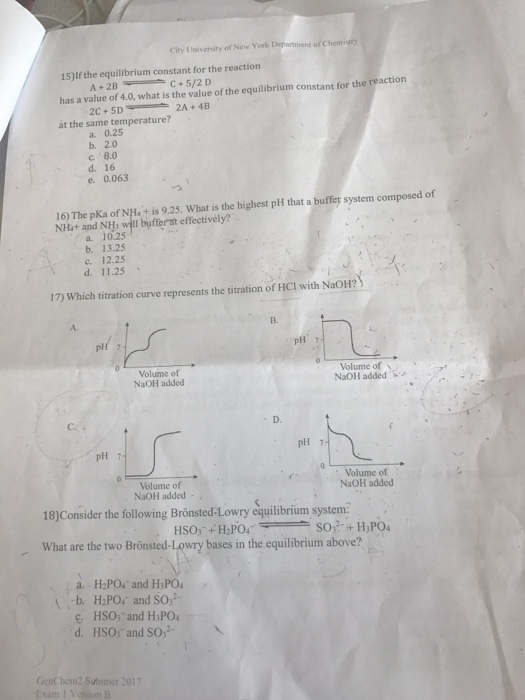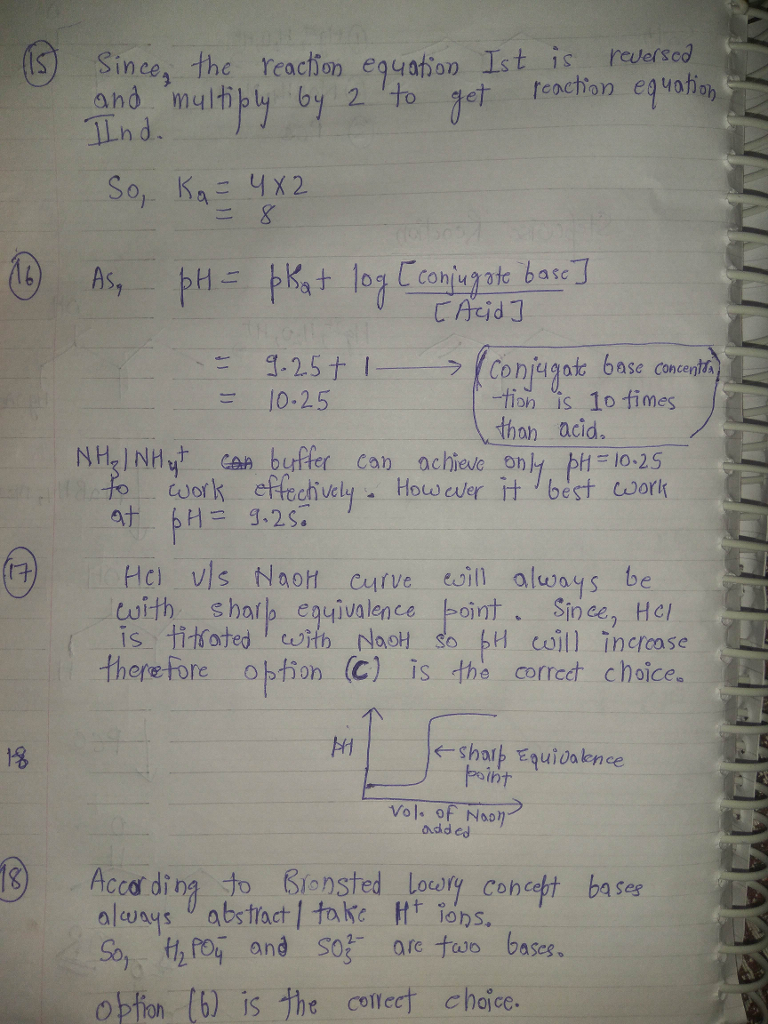# Question & Answer: City University of New York Department of Chemistry 15)If the equilibrium constant…..City University of New York Department of Chemistry 15)If the equilibrium constant for the reaction has a value of 4.0, what is the value of the equilibrium constant for the reaction át the same temperature? a. 0.25 b. 2.0 c. 8.0 d. 16 e 0.063 10 The pKa of NJHa’t is 9,25, What is the highest plt that a buffer system composed of NHst and NHb will bufferat effectively? a. 10.25 b. 13.25 c. 12.25 d. 11.25 、 17) Which titration curve represents the titration of HCl with NaOH? A. B. pH 7 Volume of NaOH added Volume ofzs NaOH added CA D. pH 7 Volume of NaOH added Volume ofl NaOH added 18) Consider tem: What are the two Brönsted-Lowry bases in the equilibrium above? a. H2P0c and HPO4 b. H:PO, and SO. c. HSO, and HiPO d. HSO, and SO,2 GenC hem2 Suhimer 2017 Exam I Verion B